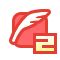# 判断一个数是否为素数或者打印某个区间的所有素数

1年前   阅读数 90 0

``````#include<stdio.h>
#include<math.h>
#include<windows.h>
#pragma warning(disable:4996)
int  IsMersenne(int n)
{
int i=0;
for(i=2;i<n;i++)//也可以这样写判断条件：i<=(int)sqrt(n)，这里的sqrt(n)意思是对数子n作开方操作；（int）意思是强制转换类型

{
if(0==n%i) //这里用n对i到n之间的所有数进行取模操作，那么用i到sqrt(n)之间可以减少循坏次数
{
return 0;//能被整除返回0，说明不是素数
}
}
return 1;//不能被整除返回1
}
int main()
{
int num=0;
scanf("%d",&num);
if(1==IsMersenne(num))
{
printf("IS Mersenne number!\n");
}
else{
printf("not Mersenne number!\n");
}
system("pause");
return 0;
}

````````````#include<stdio.h>
#include<math.h>
#include<windows.h>
#pragma warning(disable:4996)
//以打印100到200之间的素数为例。
int  IsMersenne(int n)
{
int i=0;
for(i=2;i<n;i++)//也可以这样写判断条件：i<=(int)sqrt(n)，这里的sqrt(n)意思是对数子n作开方操作；（int）意思是强制转换类型

{
if(0==n%i) //这里用n对i到n之间的所有数进行取模操作，那么用i到sqrt(n)之间可以减少循坏次数
{
return 0;//能被整除返回0，说明不是素数
}
}
return 1;//不能被整除返回1
}
int main()
{
int i=0;
for(i=100;i<200;i++)
{
if(IsMersenne(i)==1)
{
printf("%d ",i);
}
}
printf("\n");
system("pause");
return 0;
}

``````na小子叫坚强我有话说: